Search by Topic

Resources tagged with Visualising similar to Squash:

Filter by: Content type:
Age range:
Challenge level:

There are 101 results

Broad Topics > Using, Applying and Reasoning about Mathematics > VisualisingStonehenge

Age 16 to 18 Challenge Level:

Explain why, when moving heavy objects on rollers, the object moves twice as fast as the rollers. Try a similar experiment yourself.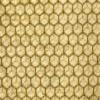Fitting Flat Shapes

Age 16 to 18 Challenge Level:

How efficiently can various flat shapes be fitted together?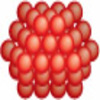Packing 3D Shapes

Age 14 to 16 Challenge Level:

What 3D shapes occur in nature. How efficiently can you pack these shapes together?Problem Solving, Using and Applying and Functional Mathematics

Age 5 to 18 Challenge Level:

Problem solving is at the heart of the NRICH site. All the problems give learners opportunities to learn, develop or use mathematical concepts and skills. Read here for more information.Mach Attack

Age 16 to 18 Challenge Level:

Have you got the Mach knack? Discover the mathematics behind exceeding the sound barrier.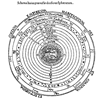Shaping the Universe II - the Solar System

Age 11 to 16

The second in a series of articles on visualising and modelling shapes in the history of astronomy.Shaping the Universe I - Planet Earth

Age 11 to 16

This article explores ths history of theories about the shape of our planet. It is the first in a series of articles looking at the significance of geometric shapes in the history of astronomy.Circuit Training

Age 14 to 16 Challenge Level:

Mike and Monisha meet at the race track, which is 400m round. Just to make a point, Mike runs anticlockwise whilst Monisha runs clockwise. Where will they meet on their way around and will they ever. . . .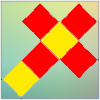Classic Cube

Age 16 to 18 Challenge Level:

The net of a cube is to be cut from a sheet of card 100 cm square. What is the maximum volume cube that can be made from a single piece of card?Bent Out of Shape

Age 14 to 18 Challenge Level:

An introduction to bond angle geometry.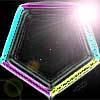Penta Colour

Age 14 to 16 Challenge Level:

In how many different ways can I colour the five edges of a pentagon red, blue and green so that no two adjacent edges are the same colour?Fermat's Poser

Age 14 to 16 Challenge Level:

Find the point whose sum of distances from the vertices (corners) of a given triangle is a minimum.Sliced

Age 14 to 16 Challenge Level:

An irregular tetrahedron has two opposite sides the same length a and the line joining their midpoints is perpendicular to these two edges and is of length b. What is the volume of the tetrahedron?Age 16 to 18 Challenge Level:

A box of size a cm by b cm by c cm is to be wrapped with a square piece of wrapping paper. Without cutting the paper what is the smallest square this can be?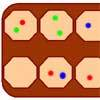Wari

Age 14 to 16 Challenge Level:

This is a simple version of an ancient game played all over the world. It is also called Mancala. What tactics will increase your chances of winning?Sliding Puzzle

Age 11 to 16 Challenge Level:

The aim of the game is to slide the green square from the top right hand corner to the bottom left hand corner in the least number of moves.Square It

Age 11 to 16 Challenge Level:

Players take it in turns to choose a dot on the grid. The winner is the first to have four dots that can be joined to form a square.Inside Out

Age 14 to 16 Challenge Level:

There are 27 small cubes in a 3 x 3 x 3 cube, 54 faces being visible at any one time. Is it possible to reorganise these cubes so that by dipping the large cube into a pot of paint three times you. . . .Cubic Net

Age 14 to 18 Challenge Level:

This is an interactive net of a Rubik's cube. Twists of the 3D cube become mixes of the squares on the 2D net. Have a play and see how many scrambles you can undo!Jam

Age 14 to 16 Challenge Level:

A game for 2 playersAll Tied Up

Age 14 to 16 Challenge Level:

A ribbon runs around a box so that it makes a complete loop with two parallel pieces of ribbon on the top. How long will the ribbon be?Something in Common

Age 14 to 16 Challenge Level:

A square of area 3 square units cannot be drawn on a 2D grid so that each of its vertices have integer coordinates, but can it be drawn on a 3D grid? Investigate squares that can be drawn.One and Three

Age 14 to 16 Challenge Level:

Two motorboats travelling up and down a lake at constant speeds leave opposite ends A and B at the same instant, passing each other, for the first time 600 metres from A, and on their return, 400. . . .Tied Up

Age 14 to 16 Short Challenge Level:

How much of the field can the animals graze?3D Treasure Hunt

Age 14 to 18 Challenge Level:

Some treasure has been hidden in a three-dimensional grid! Can you work out a strategy to find it as efficiently as possible?Changing Places

Age 14 to 16 Challenge Level:

Place a red counter in the top left corner of a 4x4 array, which is covered by 14 other smaller counters, leaving a gap in the bottom right hand corner (HOME). What is the smallest number of moves. . . .Corridors

Age 14 to 16 Challenge Level:

A 10x10x10 cube is made from 27 2x2 cubes with corridors between them. Find the shortest route from one corner to the opposite corner.Triangles Within Triangles

Age 14 to 16 Challenge Level:

Can you find a rule which connects consecutive triangular numbers?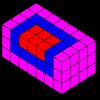Summing Squares

Age 14 to 16 Challenge Level:

Discover a way to sum square numbers by building cuboids from small cubes. Can you picture how the sequence will grow?Double Trouble

Age 14 to 16 Challenge Level:

Simple additions can lead to intriguing results...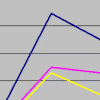Charting More Success

Age 11 to 16 Challenge Level:

Can you make sense of the charts and diagrams that are created and used by sports competitors, trainers and statisticians?Steel Cables

Age 14 to 16 Challenge Level:

Some students have been working out the number of strands needed for different sizes of cable. Can you make sense of their solutions?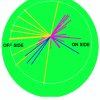Charting Success

Age 11 to 16 Challenge Level:

Can you make sense of the charts and diagrams that are created and used by sports competitors, trainers and statisticians?Triangles in the Middle

Age 11 to 18 Challenge Level:

This task depends on groups working collaboratively, discussing and reasoning to agree a final product.Mystic Rose

Age 14 to 16 Challenge Level:

Use the animation to help you work out how many lines are needed to draw mystic roses of different sizes.Speeding Boats

Age 14 to 16 Challenge Level:

Two boats travel up and down a lake. Can you picture where they will cross if you know how fast each boat is travelling?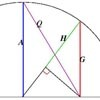Classical Means

Age 16 to 18 Challenge Level:

Use the diagram to investigate the classical Pythagorean means.Triangles Within Squares

Age 14 to 16 Challenge Level:

Can you find a rule which relates triangular numbers to square numbers?Ford Circles

Age 16 to 18 Challenge Level:

Can you find the link between these beautiful circle patterns and Farey Sequences?Baravelle

Age 7 to 16 Challenge Level:

What can you see? What do you notice? What questions can you ask?Air Nets

Age 7 to 18 Challenge Level:

Can you visualise whether these nets fold up into 3D shapes? Watch the videos each time to see if you were correct.Efficient Packing

Age 14 to 16 Challenge Level:

How efficiently can you pack together disks?Clocking Off

Age 7 to 16 Challenge Level:

I found these clocks in the Arts Centre at the University of Warwick intriguing - do they really need four clocks and what times would be ambiguous with only two or three of them?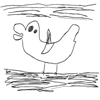The Development of Spatial and Geometric Thinking: 5 to 18

Age 5 to 16

This is the first article in a series which aim to provide some insight into the way spatial thinking develops in children, and draw on a range of reported research. The focus of this article is the. . . .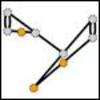Sprouts

Age 7 to 18 Challenge Level:

A game for 2 people. Take turns joining two dots, until your opponent is unable to move.Building Gnomons

Age 14 to 16 Challenge Level:

Build gnomons that are related to the Fibonacci sequence and try to explain why this is possible.LOGO Challenge - Triangles-squares-stars

Age 11 to 16 Challenge Level:

Can you recreate these designs? What are the basic units? What movement is required between each unit? Some elegant use of procedures will help - variables not essential.Around and Back

Age 14 to 16 Challenge Level:

A cyclist and a runner start off simultaneously around a race track each going at a constant speed. The cyclist goes all the way around and then catches up with the runner. He then instantly turns. . . .Vanishing Point

Age 14 to 18 Challenge Level:

How can visual patterns be used to prove sums of series?Prime Magic

Age 7 to 16 Challenge Level:

Place the numbers 1, 2, 3,..., 9 one on each square of a 3 by 3 grid so that all the rows and columns add up to a prime number. How many different solutions can you find?